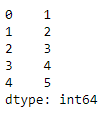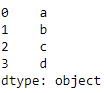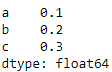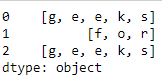# Create and display a one-dimensional array-like object using Pandas in Python

Series() is a function present in the Pandas library that creates a one-dimensional array and can hold any type of objects or data in it. In this article, let us learn the syntax, create and display one-dimensional array-like object containing an array of data using Pandas library.

## pandas.Series()

Syntax : pandas.Series(parameters)
Parameters :

• data : Contains data stored in Series.
• index : Values must be hashable and have the same length as data.
• dtype : Data type for the output Series.
• name : The name to give to the Series.
• copy : Copy input data.

Returns : An object of class Series

Example 1 : Creating Series from a list

 `# import the library ` `import` `pandas as pd ` ` `  `# create the one-dimensional array ` `data ``=` `[``1``, ``2``, ``3``, ``4``, ``5``] ` ` `  `# create the Series ` `ex1 ``=` `pd.Series(data) ` ` `  `# displaying the Series ` `print``(ex1) `

Output :Example 2 :Creating a Series from a NumPy array.

 `# import the pandas and numpy library ` `import` `pandas as pd ` `import` `numpy as np ` ` `  `# create numpy array ` `data ``=` `np.array([``'a'``, ``'b'``, ``'c'``, ``'d'``]) ` ` `  `# create one-dimensional data ` `s ``=` `pd.Series(data) ` ` `  `# display the Series ` `print``(s) `

Output :Example 3: Creating a Series from a dictionary.

 `# import the pandas library ` `import` `pandas as pd ` ` `  `# create dictionary ` `dict` `=` `{``'a'` `: ``0.1``, ``'b'` `: ``0.2``, ``'c'` `: ``0.3``} ` ` `  `# create one-dimensional data ` `s ``=` `pd.Series(``dict``) ` ` `  `# display the Series ` `print``(s) `

Output :Example 4 :Creating a Series from list of lists.

 `# importing the module ` `import` `pandas as pd ` ` `  `# creating the data ` `data ``=` `[[``'g'``, ``'e'``, ``'e'``, ``'k'``, ``'s'``], ` `        ``[``'f'``, ``'o'``, ``'r'``], ` `        ``[``'g'``, ``'e'``, ``'e'``, ``'k'``, ``'s'``]] ` ` `  `# creating a Pandas series of lists ` `s ``=` `pd.Series(data) ` ` `  `# displaying the Series ` `print``(s) `

Output :Whether you're preparing for your first job interview or aiming to upskill in this ever-evolving tech landscape, GeeksforGeeks Courses are your key to success. We provide top-quality content at affordable prices, all geared towards accelerating your growth in a time-bound manner. Join the millions we've already empowered, and we're here to do the same for you. Don't miss out - check it out now!